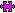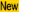Calculus Text Puzzles

Peter Jipsen, Chapman University, August 2002

This is the home page for Calculus Text Puzzles: An interactive way of reading scrambled calculus definitions, examples and proofs. Some parts of the text on these dynamic webpages can be rearranged easily, and the reader is given automatic feedback on whether the original correct form of the text has been reconstructed.

Click on some of the links below and see how this approach allows you to (re)discover concepts. This can help with understanding calculus, and it is more interesting than simply reading the textbook. It is meant as an intermediate step between studying existing definitions and proofs versus writing your own. You are encouraged to print out the unscrambled version of a completed puzzle and keep it as a study-aid. The pages below require a fairly recent web browser such as Internet Explorer 5.5+ or Netscape 6+. If you have an older browser, you may be able to view the previous version of Calculus Text Puzzles.

Instructions: Some pieces of the definitions and results below have been shuffled, and your task is to sort them into the logically correct order (if there seem to be several correct orders, choose one that produces the 'most sensible' result). Click apuzzle piece to select it, then click anotherpuzzle piece to move (or swap) the selected piece to that particular position. When you think you are done, click on the Check button. If you are happy with your grade, you can enter your name and print the page. Don't worry, the grade and your name are not recorded anywhere on the web (the Text Puzzles run locally in your browser).

Definitions and results about calculus (based on Stewarts Calculus, 4th edition).

Preliminaries: Appendix A

Definitions: Integers, Rational, Irrational and Real Numbers.
Definitions: Basic set notation.
Definitions: Intervals, open, closed, half-open.

Chapter 1: Functions and Models

Definitions: Functions, domain, range, symmetry, increasing, decreasing
Definitions: Linear, Polynomial, Power, Rational, Algebraic, Trigonometric, Exponential, Logarithmic, Transcendental Functions
Results: Shifting, stretching and reflecting functions
Exercise: Composition of two functions

Chapter 2: Limits, Continuity and Rates of Change

Discussion: Informal and formal definition of a limit.Examples: Limits of the form 0/0.Definitions: Limits, from the left and right, and to infinity.
Proposition:Proposition:Results : Limit laws.

Here are some Proof Puzzles where you can discover why the limit laws follow from the definition of limits.
Sum of Limits Theorem:
Constant Multiple of Limits Theorem:Product of Limits Theorem:
Reciprocal of Limits Theorem:
Left-Right Limit Theorem:
Limit Order Theorem:
Squeeze Theorem:

Chapter 3: Derivatives

Definitions: The Derivative at a point and as a function.(for Netscape 7 or IE+MathPlayer)
Theorem:Results: The Rules of Differentiation.(for Netscape 7 or IE+MathPlayer)
Examples: Differentiation.(for Netscape 7 or IE+MathPlayer)

Here are the proofs of the rules.
Power Law:(for Netscape 7 or IE+MathPlayer)
Constant Multiple Law:Sum Law:Difference Law:
Product Law:Quotient Law:(for Netscape 7 or IE+MathPlayer)

Chapter 4: Applications of Derivatives

Definitions: Concepts of derivatives related to finding maxima and minima.(for Netscape 7 or IE+MathPlayer)
Results: The Mean Value Theorem and some of its consequences.(for Netscape 7 or IE+MathPlayer)